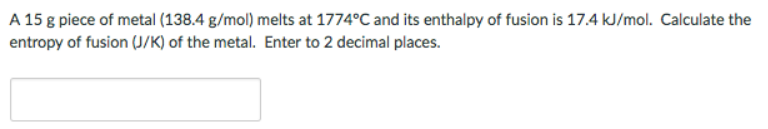# Problem: A 15 g piece of metal (138.4 g/mol) melts at 1774 °C and its enthalpy of fusion is 17.4/mol. Calculate the entropy of fusion (J/K) of the metal. Enter to 2 decimal places.

###### FREE Expert Solution
80% (290 ratings)###### Problem Details

A 15 g piece of metal (138.4 g/mol) melts at 1774 °C and its enthalpy of fusion is 17.4/mol. Calculate the entropy of fusion (J/K) of the metal. Enter to 2 decimal places.